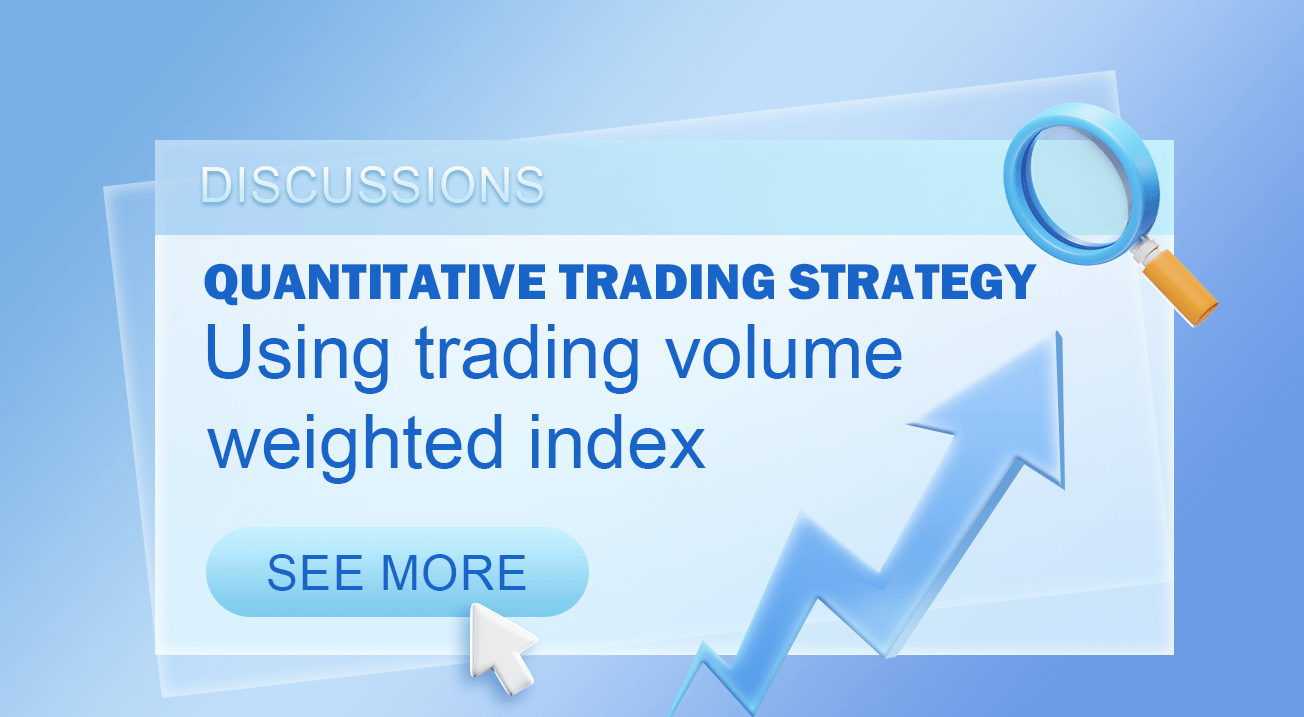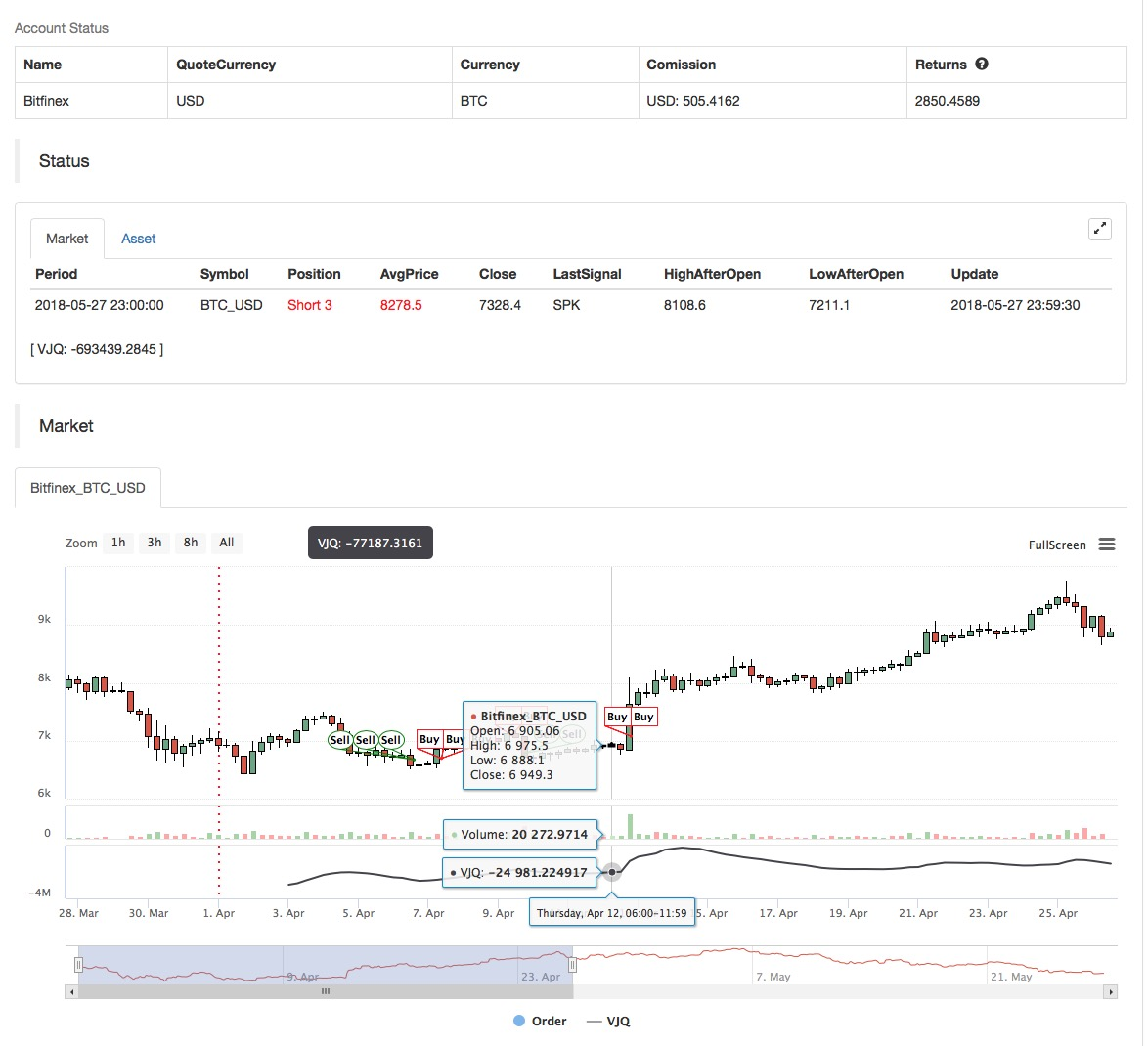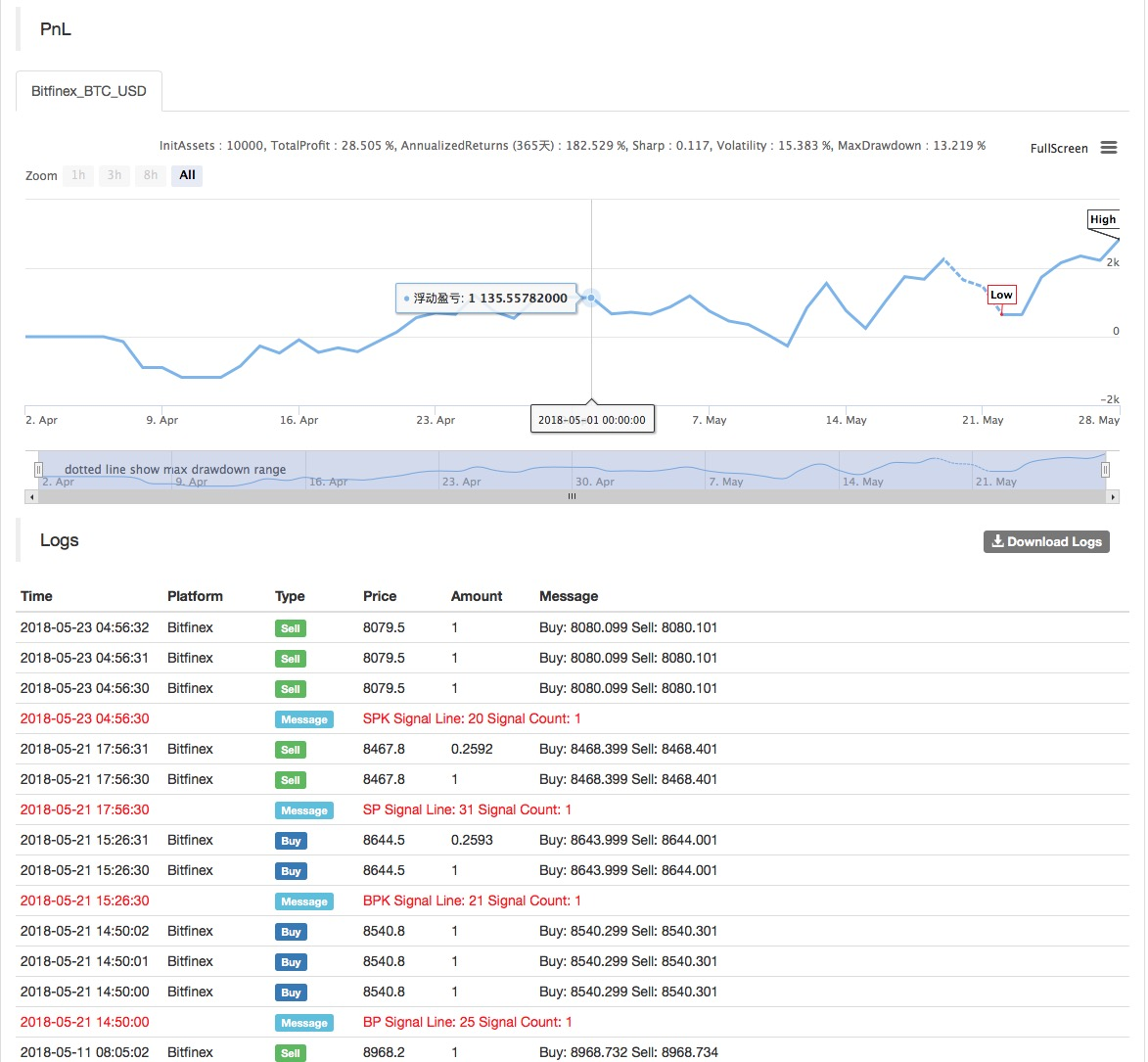Author: Lydia, Created: 2023-01-18 14:06:08, Updated: 2023-09-20 10:14:56### What is the trading volume weighted index?

The volume weighted index is an investment index, in which each investment affects the index in proportion to the trading volume of each of its investment target. Add the trading volume of each investment in the index and divide it by the total investment to determine the value of the index. The investment with higher trading volume will give more weight than the investment with lower trading volume, so it will be more indicative of the performance of the index.

### Detailed explanation of trading volume weighted index

In the trading volume weighted index, the impact of investments from \$110 to \$120 on the index will be greater than those from \$10 to \$20. Even if these increases are not as large as those at low prices, investments with high trading volume will have a greater impact on the index or the overall direction.

For example, the Dow Jones Industrial Average (DIJA) is one of the most popular trading volume weighted investments, consisting of 30 different investments or components. In this index, the trading volume of the investment target with high trading volume is higher than that of the investment target with low trading volume, so it is called trading volume weighted assignment.

### Other weighted indexes

In addition to the trading volume weighted index, other basic types of weighted indexes include value weighted index and unweighted index. For value weighted indexes, such as those in the MSCI strategic index series, the number of issued investment targets is a factor. In order to determine the weight of each investment target in the value weighted index, the trading volume of the investment target is multiplied by the number of issued investment targets. For example, if Investment A has 5,000,000 shares issued and the trading volume is \$15, its weight in the index is \$750,000,000. If the trading volume of Investment B is \$30, but only 1,000,000 shares have been issued, its weight is \$30,000,000. Therefore, in the value weighted index, Investment A will be more indicative of how the index changes than that of Investment B.

In the unweighted index, all investments have the same impact on the index, regardless of their investment volume or trading volume. Any volume change in the index is based on the return percentage of each component. For example, if Investment A increases by 30%, Investment B increases by 20%, Investment C increases by 10%, and the index increases by 20%, or 30+20+10/3, that is, the number of investment targets in the index.

Another type of weighted index is the market value weighted index, in which the share of each investment target is based on the market value of the issued investment target. Other types of weighted indexes include income weighting, basic weighting, and floating adjustment. According to investors’ goals and market perceptions, there are both positive and negative aspects.

### Application of trading volume weighted index strategy on FMZ Quant platform

Based on the above basic concepts, we have a general understanding of the basic principle and operation mechanism of this strategy. Next, we will deploy it to the FMZ Quant platform to implement this strategy in the digital currency market. We still use the easy MyLanguage for programming.

• Data period: multiple periods
• Back-testing target: OKEX futures
• Contract type: this_week``````MAN^^MA(C,N);
B_MA:=C>MAN;
S_MA:=C<MAN;

S_K1:=SUM((H-C)*V,N)/SUM((H-L)*V,N)>0.5;
B_K1:=SUM((C-L)*V,N)/SUM((H-L)*V,N)>0.5;

CO:=IF(C>O,C-O,0);
OC:=IF(C<O,O-C,0);
S_K2:=SUM(OC*V,N)/SUM(ABS(C-O)*V,N)>0.5;
B_K2:=SUM(CO*V,N)/SUM(ABS(C-O)*V,N)>0.5;

B_K1 AND B_K2 AND B_MA AND H>=HHV(H,N),BPK;
S_K1 AND S_K2 AND S_MA AND L<=LLV(L,N),SPK;

STOPLOSS:=M*MA(H-L,N);
C<BKPRICE-STOPLOSS,SP(BKVOL);
C>SKPRICE+STOPLOSS,BP(SKVOL);

S_MA AND BKHIGH>BKPRICE+STOPLOSS,SP(BKVOL);
B_MA AND SKLOW<SKPRICE-STOPLOSS,BP(SKVOL);
``````

For the strategy source code, please refer to: https://www.fmz.com/strategy/128125.

Related

More